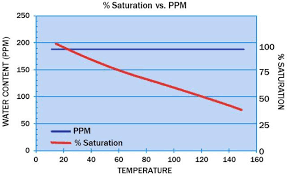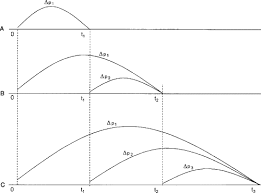## How to Calculate and Solve for Oil Saturation of Reservoir Fluid Flow | The Calculator EncyclopediaThe image above represents the oil saturation.

To compute for the oil saturation, five essential parameters are needed and these parameters are cumulative oil production (Np), oil in place (N), initial water saturation (Swi), initial oil FVF (Boi) and oil FVF (Bo).

The formula for calculating oil saturation:

S = [1 – Swi] [1 – Np / N] [Bo / Boi]

Where;

S = Oil Saturation
Np = Cumulative Oil Production
N = Oil in Place
Swi = Initial Water Saturation
Boi = Initial Oil FVF
Bo = Oil FVF

Let’s solve an example;
Given that the cumulative oil production is 22, oil in place is 8, initial water saturation is 16, initial oil FVF is 12 and the oil FVF is 7.
Find the oil saturation?

This implies that;

Np = Cumulative Oil Production = 22
N = Oil in Place = 8
Swi = Initial Water Saturation = 16
Boi = Initial Oil FVF = 12
Bo = Oil FVF = 7

S = [1 – Swi] [1 – Np / N] [Bo / Boi]
S = [1 – 16] [1 – 22/8] [7/12]
S = [1 – 16] [1 – 22/8] [0.583]
S = [1 – 16] [1 – 2.75] [0.583]
S = [1 – 16] [-1.75] [0.583]
S = [-15] [-1.75] [0.583]
S = 15.31

Therefore, the oil saturation is 15.31.

## How to Calculate and Solve for Total Compressibility of a Reservoir Fluid Flow | The Calculator EncyclopediaThe image above represents the total compressibility.

To compute for the total compressibility, seven essential parameters are needed and these parameters are Water compressibility (Cw), Oil compressibility (Co), Gas compressibility (Cg), Rock compressibility (Cr), water saturation (Sw), Oil saturation (So) and Gas saturation (Sg).

The formula for calculating the total compressibility:

CT = CoSo + CgSg + CwSw + Cr

Where;

CT = Total Compressibility
Cw = Water Compressibility
Co = Oil Compressibility
Cg = Gas Compressibility
Cr = Rock Compressibility
Sw = Water Saturation
So = Oil Saturation
Sg = Gas Saturation

Let’s solve an example;
With a given value of 13 as water compressibility, 17 as oil compressibility, 19 as gas compressibility, 25 as rock compressibility, 24 as water saturation, 29 as oil saturation and 21 as gas saturation.
Find the total compressibility?

This implies that;

Cw = Water Compressibility = 13
Co = Oil Compressibility = 17
Cg = Gas Compressibility = 19
Cr = Rock Compressibility = 25
Sw = Water Saturation = 24
So = Oil Saturation = 29
Sg = Gas Saturation = 21

CT = CoSo + CgSg + CwSw + Cr
CT = (17 x 29) + (19 x 21) + (13 x 24) + 25
CT = 493 + 399 + 312 + 25
CT = 1229

Therefore, the total compressibility is 1229 psi.